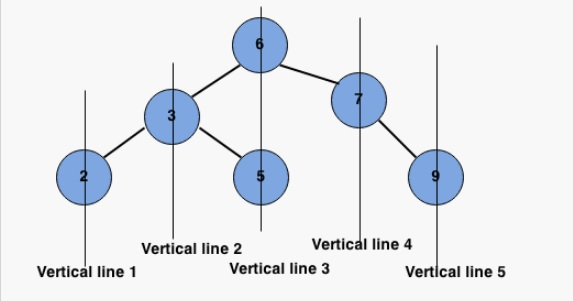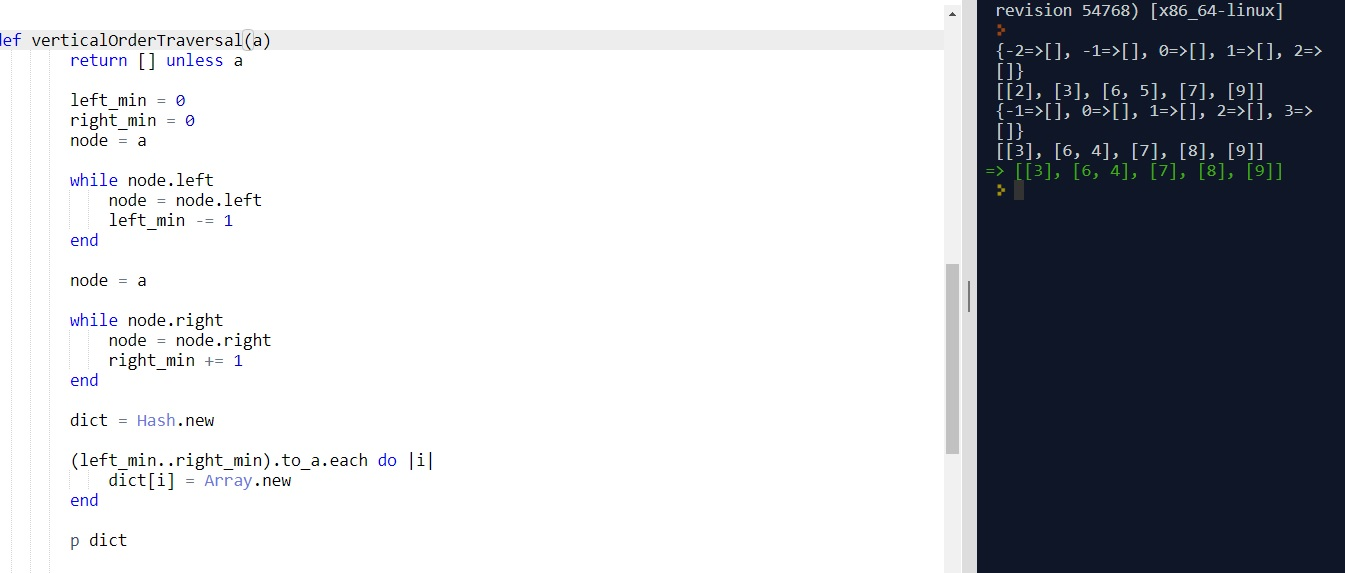# VERTICAL ORDER TRAVERSAL OF BINARY TREE

By: Saurav

2018-01-15 23:20:00 UTC

Given a binary tree, print a vertical order traversal of it.

Example :
Given binary tree:

6
/ \
3 7
/ \ \
2 5 9
returns

[
,
,
[6 5],
,

]

InterviewBit

At first glance, this problem looks a little intimidating. We have had DFS, BFS, recursion till the leaf, level order etc but how will you deal with a vertical order traversal like the one shown below:Attempt 1:

The Idea is to use a method of finding the width of the tree by fixing the root at position 0. The length is the leftmost (-ve) index + rightmost index.

So, first, we need to find the maximum width (horizontally) of the tree.
Then, we need to assign a hash map with ranges between leftmost and rightmost indices (inclusive) and allot an array as each value to put the nodes correspondingly.
Finally, we need to traverse the tree in recursion and allot each node to the respective value array. For a given node, its relative value is parent's value - 1 if its left child, or else it parent + 1 if its a right child.

Let's see the code in action:

```def verticalOrderTraversal(a)
return [] unless a

left_min = 0
right_min = 0
node = a

while node.left
node = node.left
left_min -= 1
end

node = a

while node.right
node = node.right
right_min += 1
end

dict = Hash.new

(left_min..right_min).to_a.each do |i|
dict[i] = Array.new
end

add_to_respective_array(a, 0, dict)

final = dict.map{|key, value| value}

return final
end

def add_to_respective_array(node, value, dict)
return unless node

dict[value] << node.value

if node.left
add_to_respective_array(node.left, value-1, dict)
end
if node.right
add_to_respective_array(node.right, value+1, dict)
end

return dict
end```

Running the code with the tests (I took a BST, but we can use a BT as well):```bst = BST.new
root =  bst.insert(6, bst.root)
root = bst.insert(3, root)
root = bst.insert(2, root)
root = bst.insert(5, root)
root = bst.insert(7, root)
root = bst.insert(9, root)
p verticalOrderTraversal(root)

bst = BST.new
root =  bst.insert(6, bst.root)
root = bst.insert(7, root)
root = bst.insert(8, root)
root = bst.insert(9, root)
root = bst.insert(3, root)
root = bst.insert(4, root)
p verticalOrderTraversal(root)```

Looks good to me!

### Let me know what you think! Twitter: sprakash24octLinkedin

Owned & Maintained by Saurav Prakash

If you like what you see, you can help me cover server costs or buy me a cup of coffee though donation :)